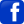﻿ Tonal Analysis Report - page 1UK Registered Charity Number: 1154107Conservation by Re-use

Helping churches acquire surplus and/or redundant bells to be hung for

English-style full-circle bell-ringing.Index Previous page Next pageSound of Bells – Tonal Analysis Report

The table shows a typical tonal analysis report for a ring of eight bells. It gives the Note of each bell, the frequency of the five main partials, the errors of individual bells and of the entire ring.

Tonal analysis report for a ring of bells

The table comprises of eight columns, one for each bell in the ring. Each column is split into six rows; the top column is the bell’s Note and the remaining columns are the five main partials which make the sound of the bell: the Nominal, Hum, Prime, Tierce and Quint. The upper two rows give an indication of how in-tune each bell is within the ring. It is usual to use the tenor bell’s Nominal partial as the datum for the ring of bells. The lower four rows (Hum, Prime, Tierce and Quint) give an indication of the tuning of each individual bell; the bell’s Nominal partial is used as the datum.

The bell Note is usually given using the Standard International Pitch of A = 440 Hz. The Nominal : frequency Hz row gives the same information - but as a frequency instead of a Note. The Nominal : Ratio gives the ratio of each bell and the datum's nominal frequency – usually the tenor bell‘s Nominal frequency. The Equal Tempered scale is normally used for these ratios, but other tuning standards may be used. The Nominal : Error gives the error or difference (in cents) between the bell’s actual frequency and the theoretical Equal Tempered scale frequency. For example the 7th bell has a note of F#-47 cents and is therefore 22 cents flat of the ideal note of F#-25 cents. Likewise the treble is 17 cents sharp of the expected note of E-25. The last four rows give the frequency of the remaining partials, the ratio of the bell's partial to its nominal frequency and the difference or error (in cents) between the actual and expected frequency for a Simpson tuned bell. The ratios for Simpson tuning are: Hum  : 0.25, Prime : 0.50, Tierce : 0.60 and Quint :  0.75.